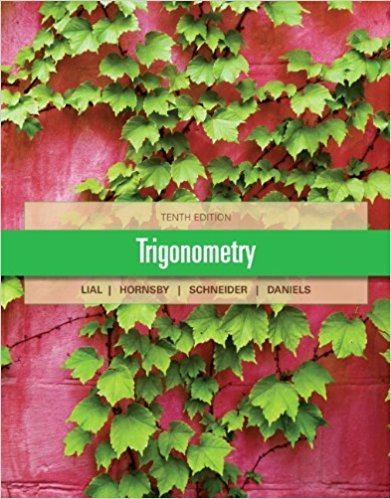×
Log in to StudySoup
Get Full Access to Math - Textbook Survival Guide
Join StudySoup for FREE
Get Full Access to Math - Textbook Survival Guide

Already have an account? Login here
×
Reset your password

Textbooks / Math / Trigonometry 10

# Trigonometry 10th Edition - Solutions by Chapter## Full solutions for Trigonometry | 10th Edition

ISBN: 9780321671776Trigonometry | 10th Edition - Solutions by Chapter

Solutions by Chapter
4 5 0 282 Reviews
##### ISBN: 9780321671776

The full step-by-step solution to problem in Trigonometry were answered by , our top Math solution expert on 01/11/18, 01:35PM. This expansive textbook survival guide covers the following chapters: 59. Trigonometry was written by and is associated to the ISBN: 9780321671776. Since problems from 59 chapters in Trigonometry have been answered, more than 56168 students have viewed full step-by-step answer. This textbook survival guide was created for the textbook: Trigonometry, edition: 10.

Key Math Terms and definitions covered in this textbook
• Adjacency matrix of a graph.

Square matrix with aij = 1 when there is an edge from node i to node j; otherwise aij = O. A = AT when edges go both ways (undirected). Adjacency matrix of a graph. Square matrix with aij = 1 when there is an edge from node i to node j; otherwise aij = O. A = AT when edges go both ways (undirected).

• Block matrix.

A matrix can be partitioned into matrix blocks, by cuts between rows and/or between columns. Block multiplication ofAB is allowed if the block shapes permit.

• Cayley-Hamilton Theorem.

peA) = det(A - AI) has peA) = zero matrix.

• Column space C (A) =

space of all combinations of the columns of A.

• Complete solution x = x p + Xn to Ax = b.

(Particular x p) + (x n in nullspace).

• Factorization

A = L U. If elimination takes A to U without row exchanges, then the lower triangular L with multipliers eij (and eii = 1) brings U back to A.

• Fibonacci numbers

0,1,1,2,3,5, ... satisfy Fn = Fn-l + Fn- 2 = (A7 -A~)I()q -A2). Growth rate Al = (1 + .J5) 12 is the largest eigenvalue of the Fibonacci matrix [ } A].

• Fourier matrix F.

Entries Fjk = e21Cijk/n give orthogonal columns FT F = nI. Then y = Fe is the (inverse) Discrete Fourier Transform Y j = L cke21Cijk/n.

• Free variable Xi.

Column i has no pivot in elimination. We can give the n - r free variables any values, then Ax = b determines the r pivot variables (if solvable!).

• Identity matrix I (or In).

Diagonal entries = 1, off-diagonal entries = 0.

• Krylov subspace Kj(A, b).

The subspace spanned by b, Ab, ... , Aj-Ib. Numerical methods approximate A -I b by x j with residual b - Ax j in this subspace. A good basis for K j requires only multiplication by A at each step.

• lA-II = l/lAI and IATI = IAI.

The big formula for det(A) has a sum of n! terms, the cofactor formula uses determinants of size n - 1, volume of box = I det( A) I.

• Left inverse A+.

If A has full column rank n, then A+ = (AT A)-I AT has A+ A = In.

• Markov matrix M.

All mij > 0 and each column sum is 1. Largest eigenvalue A = 1. If mij > 0, the columns of Mk approach the steady state eigenvector M s = s > O.

• Multiplication Ax

= Xl (column 1) + ... + xn(column n) = combination of columns.

• Pivot.

The diagonal entry (first nonzero) at the time when a row is used in elimination.

• Reflection matrix (Householder) Q = I -2uuT.

Unit vector u is reflected to Qu = -u. All x intheplanemirroruTx = o have Qx = x. Notice QT = Q-1 = Q.

• Semidefinite matrix A.

(Positive) semidefinite: all x T Ax > 0, all A > 0; A = any RT R.

• Similar matrices A and B.

Every B = M-I AM has the same eigenvalues as A.

• Spectrum of A = the set of eigenvalues {A I, ... , An}.

Spectral radius = max of IAi I.